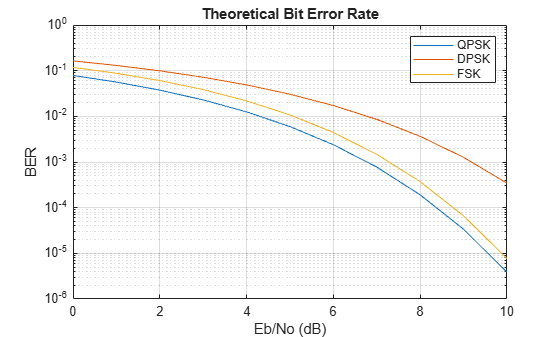# berawgn

Bit error rate (BER) for uncoded AWGN channels

## Syntax

```ber = berawgn(EbNo,'pam',M) ber = berawgn(EbNo,'qam',M) ber = berawgn(EbNo,'psk',M,dataenc) ber = berawgn(EbNo,'oqpsk',dataenc) ber = berawgn(EbNo,'dpsk',M) ber = berawgn(EbNo,'fsk',M,coherence) ber = berawgn(EbNo,'fsk',2,coherence,rho) ber = berawgn(EbNo,'msk',precoding) ber = berawgn(EbNo,'msk',precoding,coherence) berlb = berawgn(EbNo,'cpfsk',M,modindex,kmin) [BER,SER] = berawgn(EbNo, ...) ```

## Description

### For All Syntaxes

The `berawgn` function returns the BER of various modulation schemes over an additive white Gaussian noise (AWGN) channel. The first input argument, `EbNo`, is the ratio of bit energy to noise power spectral density, in dB. If `EbNo` is a vector, the output `ber` is a vector of the same size, whose elements correspond to the different Eb/N0 levels. The supported modulation schemes, which correspond to the second input argument to the function, are in the following table.

Modulation SchemeSecond Input Argument
Phase shift keying (PSK) `'psk'`
Offset quadrature phase shift keying (OQPSK)`'oqpsk'`
Differential phase shift keying (DPSK) `'dpsk'`
Pulse amplitude modulation (PAM) `'pam'`
Quadrature amplitude modulation (QAM) `'qam'`
Frequency shift keying (FSK) `'fsk'`
Minimum shift keying (MSK) `'msk'`
Continuous phase frequency shift keying (CPFSK) `'cpfsk'`

Most syntaxes also have an `M` input that specifies the alphabet size for the modulation. `M` must have the form 2k for some positive integer `k`. For all cases, the function assumes the use of a Gray-coded signal constellation.

### For Specific Syntaxes

`ber = berawgn(EbNo,'pam',M)` returns the BER of uncoded PAM over an AWGN channel with coherent demodulation.

`ber = berawgn(EbNo,'qam',M)` returns the BER of uncoded QAM over an AWGN channel with coherent demodulation. The alphabet size, `M`, must be at least 4. When $k={\mathrm{log}}_{2}M$ is odd, a rectangular constellation of size $M=I×J$ is used, where $I={2}^{\frac{k-1}{2}}$ and $J={2}^{\frac{k+1}{2}}$. When k is even, a square constellation of size${2}^{\frac{k}{2}}×{2}^{\frac{k}{2}}$ is used.

`ber = berawgn(EbNo,'psk',M,dataenc)` returns the BER of coherently detected uncoded PSK over an AWGN channel. `dataenc` is either `'diff'` for differential data encoding or `'nondiff'` for nondifferential data encoding. If `dataenc` is `'diff'`, `M` must be no greater than 4.

`ber = berawgn(EbNo,'oqpsk',dataenc)` returns the BER of coherently detected offset-QPSK over an uncoded AWGN channel.

`ber = berawgn(EbNo,'dpsk',M) ` returns the BER of uncoded DPSK modulation over an AWGN channel.

`ber = berawgn(EbNo,'fsk',M,coherence)` returns the BER of orthogonal uncoded FSK modulation over an AWGN channel. `coherence` is either `'coherent'` for coherent demodulation or `'noncoherent'` for noncoherent demodulation. `M` must be no greater than 64 for `'noncoherent'`.

`ber = berawgn(EbNo,'fsk',2,coherence,rho)` returns the BER for binary nonorthogonal FSK over an uncoded AWGN channel, where `rho` is the complex correlation coefficient. See Nonorthogonal 2-FSK with Coherent Detection for the definition of the complex correlation coefficient and how to compute it for nonorthogonal BFSK.

`ber = berawgn(EbNo,'msk',precoding)` returns the BER of coherently detected MSK modulation over an uncoded AWGN channel. Setting `precoding` to `'off'` returns results for conventional MSK while setting `precoding` to `'on'` returns results for precoded MSK.

`ber = berawgn(EbNo,'msk',precoding,coherence)` specifies whether the detection is coherent or noncoherent.

`berlb = berawgn(EbNo,'cpfsk',M,modindex,kmin) ` returns a lower bound on the BER of uncoded CPFSK modulation over an AWGN channel. `modindex` is the modulation index, a positive real number. `kmin` is the number of paths having the minimum distance; if this number is unknown, you can assume a value of 1.

`[BER,SER] = berawgn(EbNo, ...)` returns both the BER and SER.

## Examples

collapse all

This example shows how to generate theoretical bit error rate data for several modulation schemes assuming an AWGN channel.

Create a vector of Eb/No values and set the modulation order, `M`.

```EbNo = (0:10)'; M = 4;```

Generate theoretical BER data for QPSK modulation by using the `berawgn` function.

`berQ = berawgn(EbNo,'psk',M,'nondiff');`

Generate equivalent data for DPSK and FSK.

```berD = berawgn(EbNo,'dpsk',M); berF = berawgn(EbNo,'fsk',M,'coherent');```

Plot the results.

```semilogy(EbNo,[berQ berD berF]) xlabel('Eb/No (dB)') ylabel('BER') legend('QPSK','DPSK','FSK') grid```## Limitations

The numerical accuracy of this function's output is limited by approximations related to the numerical implementation of the expressions.

You can generally rely on the first couple of significant digits of the function's output.

## Alternatives

As an alternative to the `berawgn` function, invoke the BERTool GUI (`bertool`), and use the Theoretical tab.

## References

 Anderson, John B., Tor Aulin, and Carl-Erik Sundberg, Digital Phase Modulation, New York, Plenum Press, 1986.

 Cho, K., and Yoon, D., “On the general BER expression of one- and two-dimensional amplitude modulations”, IEEE Trans. Commun., Vol. 50, Number 7, pp. 1074-1080, 2002.

 Lee, P. J., “Computation of the bit error rate of coherent M-ary PSK with Gray code bit mapping”, IEEE Trans. Commun., Vol. COM-34, Number 5, pp. 488-491, 1986.

 Proakis, J. G., Digital Communications, 4th ed., McGraw-Hill, 2001.

 Simon, M. K, Hinedi, S. M., and Lindsey, W. C., Digital Communication Techniques – Signal Design and Detection, Prentice-Hall, 1995.

 Simon, M. K, “On the bit-error probability of differentially encoded QPSK and offset QPSK in the presence of carrier synchronization”, IEEE Trans. Commun., Vol. 54, pp. 806-812, 2006.

 Lindsey, W. C., and Simon, M. K, Telecommunication Systems Engineering, Englewood Cliffs, N.J., Prentice-Hall, 1973.